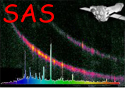XMM-Newton Science Analysis System

psfgen (psfgen-1.7) [xmmsas_20211130_0941-20.0.0]

## Example 4: Rotated PSF image

psfgen instrument=EPN energy=3000 coordtype=TEL x=600 y=1.0466 level=ELLBETA xsize=400 ysize=400
output=psf.fits rotate=162.0

As example 2 but with the PSF rotated by 162 degrees. Note that the rotation angle needed to allow comparison with a sky coordinate image would typically be PA degrees, where PA is the position angle of the observation, given by the PA_PNT keyword.

XMM-Newton SOC -- 2021-11-30